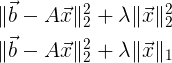# Squared norm + Regularizer

Hello,

I have 2 problems I wish to solve in CVX.
Solve x that minimizes my objective function, matrix A and vector b are known.My attempt for 1st objective function
variable x( size(A,2) );
minimize ( (b - Ax)’(b - A*x) + lambda * x’ * x );

My attempt for 2nd objective function
variable x( size(A,2) );
minimize ( (b - Ax)’(b - A*x) + lambda * norm(x,1) );

Are these correct? Is there any better way to write them in CVX?

These are correct, although there are alternative ways of writing them.

Thank you Mark.

Is there any benefit to alternative ways (like efficiency) or are they all equivalent in CVX?

Equivalent formulations for the first term: `sum_square(A*x-b)` and `square_pos(norm(A*x-b))`.
I am not sure whether there are any efficiency differences among these, but I wouldn’t worry about it. However …

If you didn’t have another term in the objective function, then the following would be equivalent in the sense of having the same argmin (other than roundoff and if there are multiple argmins).
`norm(A*x-b)`
and is preferred in CVX for numerical reasons. See http://cvxr.com/cvx/doc/advanced.html#eliminating-quadratic-forms for a discussion of all this.

Of course, you could add a squared (or non-squared) regularizer term to `norm(A*x-b)` and that would be a different (non-equivalent), but possibly meritorious, regularization

1 Like

I see from the link you provided that using norm is likely to produce more accurate results.
Like the example in the link, I wish to vary lambda to study the trade off between the residual and the regularizer.

That suggests
To solve for x, I can keep my functions the same.
To study the trade off in varying lambda, I should reformulate my functions as follows

minimize ( (b - Ax)’(b - Ax) + lambda * x’ * x );
Reformulate to
minimize ( norm(b - A
x) + lambda * x’ * x );

minimize ( (b - Ax)’(b - Ax) + lambda * norm(x,1) );
Reformulate to
minimize ( norm(b - A
x) + lambda * norm(x,1) );

As you explained, these new objective functions are non-equivalent.
For a fixed lambda, the new objective functions will yield different values of x.
But when I vary lambda, they will produce the same trade off curve.

Am I understanding this correctly?

Yes, you are understanding this correctly